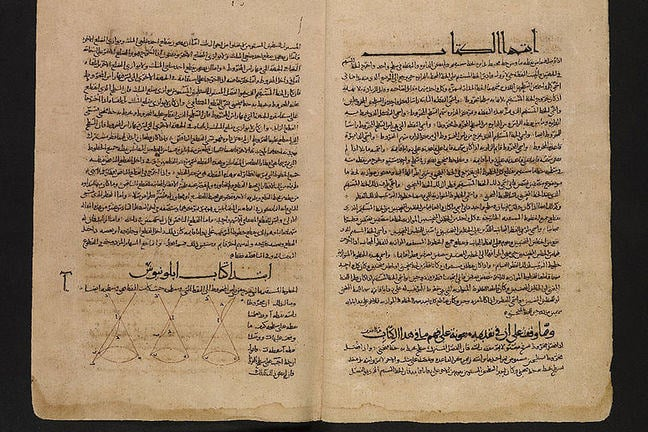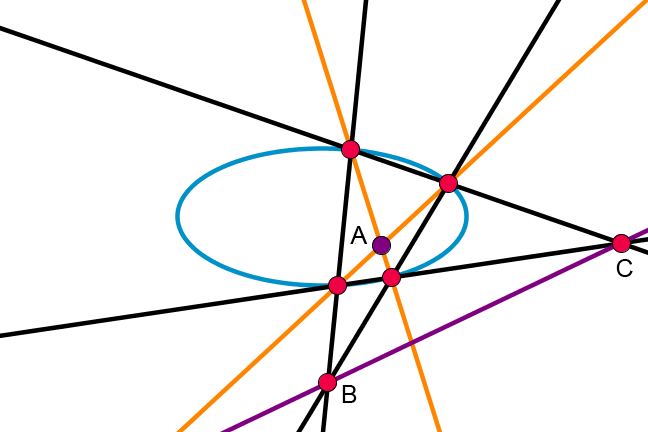3.3

## UNSW SydneyApollonius' "Conics" in Arabic

# Apollonius and conic sections

The ancient Greeks loved the simplicity and elegance of the line and the circle. In three-dimensional space, combining a circle with a fixed point not in the plane of the circle gives a cone, and it was by slicing this cone that Apollonius studied what were to become some of the most important curves in mathematics: the conic sections.

In this step, we will

• see how Apollonius defined the conic sections, or conics

• learn about several beautiful properties of conics that have been known for over 2000 years.

## Apollonius of Perga

Apollonius (262 – 190 BC) was the ancient world’s greatest geometer. He lived in Perga, which is in modern day Turkey, and wrote a series of books on conic sections, including the parabola, ellipse and hyperbola. Many of the facts discovered by him would surprise modern high school students for their elegance and richness.

The ancient Greeks regarded the line and the circle as the most fundamental and beautiful of all mathematical objects, and if you connect a circle and a point in three-dimensional space with lines, you get a cone. You might be thinking of a cone like an ice-cream cone, but Apollonius realised it is useful to think of the lines of the cone as extending in both directions — a two sided cone.

## Slicing a cone

Now if we slice such a cone with a plane, we get generally three different kinds of curves, along with a few more special cases. It all depends on the relation between the slicing plane and the cone.Conic sections” Public Doman/Wikimedia Commons

The hyperbola is the case when the plane meets both top and bottom of the cone, the ellipse is the case when we meet only one half of the cone in a finite or bounded region. The parabola is poised exactly in between the ellipse and the hyperbola; it occurs when the slicing plane is parallel to a tangent plane of the cone. What is a tangent plane? Imagine taking the top half of the cone and rolling it along a plane (but not unrolling it!). The plane along which the cone is rolled always forms a tangent plane to the cone and touches the cone along only a single line.

Q1 (E): An everyday example of a conic section is the shadow from a lamp with a cylindrical lampshade onto a flat surface. What conic section would you get from the shadow formed on the floor? How about on the wall?

Q2 (M): Actually there are a few other, more degenerate possibilities for what happens when a plane slices a cone. Can you describe these?

Apollonius knew all kinds of lovely facts about the conic sections. For example…

## Symmetry, centres and axes of ellipses and hyperbolas

The conic sections are quite symmetrical objects. The ellipse and the hyperbola both have a distinguished point of symmetry, called naturally enough the centre. If we reflect any point on the curve in this centre, we get another point on the curve. Through the centre of an ellipse or a hyperbola are two special lines of symmetry called the axes of the conic. If we reflect any point on the curve in such a line, then we get another point on the curve.

However the parabola is quite different in this respect: it does not have a centre, but it does have a line of symmetry — but only one! So we speak of the axis of a parabola, since it is unique. Note the terminology here: axis is the singular, and axes is the plural.

Q3 (E): Can you find the centre and axes of the conics pictured?## Conjugate diameters of an ellipse

A chord of a conic is a line that passes through two points on that conic, and a diameter is a chord that passes through the centre of a conic.

If you choose any line through the centre of an ellipse, for example $\normalsize{AB}$ in the figure below, and take the midpoints of all chords which are parallel to that diameter, then you get another diameter of the ellipse, namely $\normalsize{CD}$. And if you repeat the process with the second diameter, you get back the first diameter $\normalsize{AB}$. Try it yourself! So diameters of an ellipse come in pairs, called conjugate diameters.Q4 (C) Does a hyperbola also have a notion of conjugate diameters?

## The polarity defined by a conic (advanced topic)

Apollonius knew that if you took any conic section, then that curve determined a beautiful and remarkable correspondence, often called polarity, between points and lines in the plane.

There is a correspondence between a point on a curve and the tangent line to the curve. The concept of polarity generalises this correspondence to points which are not on the curve.

Refer to the figure of the ellipse below. Given a point $\normalsize{A}$, choose any two lines that pass through $\normalsize{A}$ and intersect the ellipse at two points. Now take those four points of intersection, and construct the lines passing through each pair of these points. These lines will meet each other, defining two other diagonal points, $\normalsize B$ and $\normalsize C$. The dual or polar of point $\normalsize{A}$ is then the line $\normalsize BC$ through those two diagonal points (the purple line in the diagram).

Remarkably this dual line is independent of the choices of lines through $\normalsize A$, as Apollonius realised.## What Apollonius didn’t know

Apollonius did not know everything there was to know about conics. He was also an astronomer, and interested in the ancient problem of describing the motion of the visible planets in the night sky. Wouldn’t he have been amazed to learn that two thousand years after him, the very conics that he loved so dearly would prove to be the key to unravelling this greatest scientific problem!

## Answers

A1. As long as your lamp hasn’t fallen over and your walls are at 90° to the floor, then the shadow on the floor should be a circle and the shadow on the wall will be a hyperbola.

A2. If the plane passes through the apex (point) of the cone, then we could get a pair of lines, or a single line, or just the single point of the apex itself. So these are also in some sense degenerate conics.

A3. The top-left gold point is the centre of the ellipse, the other gold point is the centre of the hyperbola, and the line not through either of those is the axis of the parabola.

A4. Yes, the hyperbola also has conjugate diameters. You can verify this yourself if have a picture of a hyperbola to play with.

## Get a taste of this course

Find out what this course is like by previewing some of the course steps before you join: